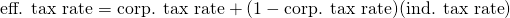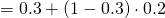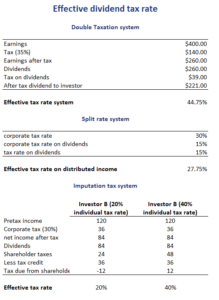# Effective dividend tax rate

The effective tax rate for dividends is different from the dividend tax rate. The reason for this is the fact that a corporation’s earnings are first taxed at the corporate level. Thus, the effective tax rate is generally higher than the statutory tax rate.

On this page, we discuss how to calculate effective tax rates for individuals. We also include an effective tax rate calculator at the bottom the page. The example shows that we can easily calculate the effective tax rate using an Excel spreadsheet.

## Effective tax rate formula

How to find the effective tax rate? First, the effective tax rate depends on the tax rate system. There are several possible methods. The most common one, which is used in the United States, is the double taxation system. This means that a corporation’s earnings are first taxed at the corporate level. Next, when the corporation pays out dividends, these are also taxed.

The effective tax rate can then be calculated using the following effective tax rate formula## Effective tax rate calculation example

Next, let’s apply a simple numerical example to illustrate how to calculate the effective rate. Let’s assume that the corporation pays a corporate tax rate of 30%. The individual investor that owns the stocks is subject to a dividend tax rate of 20%. Then we can calculate the effective dividend tax rate as followsThis method only applies under a double taxation system. In the case of a different tax system, the calculation is different. That is way we summarized the results for a number of other tax systems in the following table. These other tax systems are the split-rate corporate tax system and the imputation tax system.## Summary

We discussed how to find the effective tax rate. If the government uses a double-taxation system, we can easily find the effective tax rate using the above formula. In other cases, the tax rate can be lower if a lower corporate tax rate is used for earnings to be distributed as dividends or when the tax rate is the individual’s tax rate.